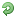##Perl Tutorial: Scalar Variables

Scalar variables

The most basic kind of variable in Perl is the scalar variable. Scalar variables hold both strings and numbers, and are remarkable in that strings and numbers are completely interchangable. For example, the statement
\$priority = 9;
sets the scalar variable \$priority to 9, but you can also assign a string to exactly the same variable:\$priority = 'high';
Perl also accepts numbers as strings, like this:\$priority = '9';
\$default = '0009';
and can still cope with arithmetic and other operations quite happily.In general variable names consists of numbers, letters and underscores, but they should not start with a number and the variable \$_ is special, as we'll see later. Also, Perl is case sensitive, so \$a and \$A are different.

Operations and Assignment

Perl uses all the usual C arithmetic operators:\$a = 1 + 2; # Add 1 and 2 and store in \$a
\$a = 3 - 4; # Subtract 4 from 3 and store in \$a
\$a = 5 * 6; # Multiply 5 and 6
\$a = 7 / 8; # Divide 7 by 8 to give 0.875
\$a = 9 ** 10; # Nine to the power of 10
\$a = 5 % 2; # Remainder of 5 divided by 2
++\$a; # Increment \$a and then return it
\$a++; # Return \$a and then increment it
--\$a; # Decrement \$a and then return it
\$a--; # Return \$a and then decrement it
and for strings Perl has the following among others:\$a = \$b . \$c; # Concatenate \$b and \$c
\$a = \$b x \$c; # \$b repeated \$c times
To assign values Perl includes\$a = \$b; # Assign \$b to \$a
\$a += \$b; # Add \$b to \$a
\$a -= \$b; # Subtract \$b from \$a
\$a .= \$b; # Append \$b onto \$a
Note that when Perl assigns a value with \$a = \$b it makes a copy of \$b and then assigns that to \$a. Therefore the next time you change \$b it will not alter \$a.Other operators can be found on the perlop manual page. Type man perlop at the prompt.

Interpolation

The following code prints apples and pears using concatenation:\$a = 'apples';
\$b = 'pears';
print \$a.' and '.\$b;
It would be nicer to include only one string in the final print statement, but the lineprint '\$a and \$b';
prints literally \$a and \$bwhich isn't very helpful. Instead we can use the double quotes in place of the single quotes:print "\$a and \$b";
The double quotes force interpolation of any codes, including interpreting variables. This is a much nicer than our original statement. Other codes that are interpolated include special characters such as newline and tab. The code\n is a newline and\t is a tab.
Exercise

This exercise is to rewrite the Hello world program so that (a) the string is assigned to a variable and (b) this variable is then printed with a newline character. Use the double quotes and don't use the concatenation operator. Make sure you can get this to work before proceeding.

› See More: Perl Tutorial: Scalar Variables